NONLINEAR PHENOMENA IN COMPLEX SYSTEMS
An Interdisciplinary Journal

2004, Vol.7, No.3, pp.250-262

Ricci Coefficients in Covariant Dirac Equation, Symmetry Aspects and Newmann-Penrose Approach.
V.M. Red'kov

The paper investigates how the Ricci rotation coefficients act in the Dirac equation in presence of external gravitational fields described in terms of Riemannian space-time geometry. It is shown that only 8 different combinations of the Ricci coefficients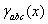are involved in the Dirac equation. They are combined in two 4-vectors Ba(x) and Ca(x) under local Lorentz group which has status of the gauge symmetry group. In all orthogonal coordinates one of these vectors, "pseudovector" Ca(x), vanishes identically. The gauge transformation laws of the two vectors are found explicitly. Connection of these Ba(x) and Aa(x) with the known Newmann-Penrose coefficients is established. General study of gauge symmetry aspects in Newmann-Penrose formalism is performed. Decomposition of the Ricci object, "tensor", into two "spinors"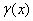and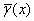is done. At this Ricci rotation coefficients are divided into two groups: 12 complex functions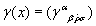and 12 conjugated to them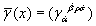. Components of spinorcoincide with 12 spin coefficients by Newmann-Penrose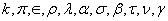. For listing these it is used a special letter-notation Li , Ni , Mi,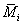. The formulas for gauge transformations of spin coefficients under local Lorentz group are derived. There are given two solutions to the gauge problem: one in the compact form of transformation laws for spinorsand, and another as detailed elaboration of the latter in terms of 12 spin coefficients.
Key words: Dirac equation, Ricci and Newmann-Penrose coefficients, gauge symmetry

Full text:  Acrobat PDF  (185KB)   PostScript (355KB)   PostScript.gz (155KB)

Copyright © Nonlinear Phenomena in Complex Systems. Last updated: November 8, 2004